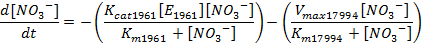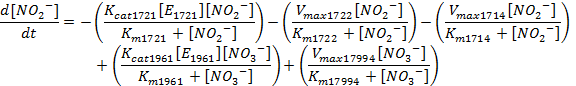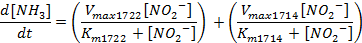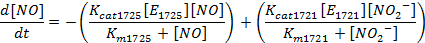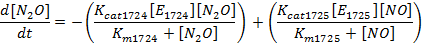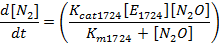# Team:NYMU-Taipei/ymim1

NYMU iGEM

Modeling

Objective

Before designing our experiment, we would like to do a simple simulation to estimate the efficiency and productivity of nitrogen for our modified bacteria.

Description

In order to produce nitrogen from nitrate, we needed to use Michaelis-Menten’s equation to help us simulate the substrate concentration in a single cell.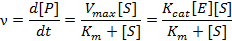Where v=reaction rate, [P]=product concentration, Vmax=max reaction rate, [S]=substrate concentration, Km= equilibrium constant of enzyme, Kcat=turnover number.

Our pathway looked like this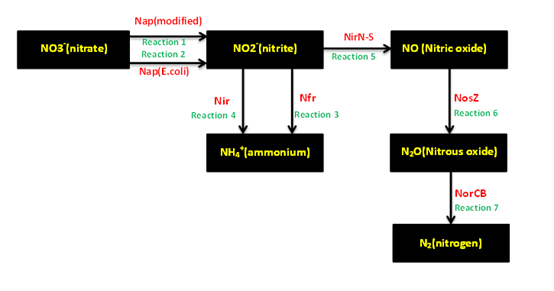Reaction 2, 3, 4 were already in the E.coli before we engineered the bacteria. Reaction 1, 5, 6, 7 are the new pathways that we engineered.

For reaction 2, 3, 4, we used the equation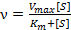As for the rest, we used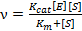instead.

To determine the enzyme concentration for our new pathways, we needed another two equations.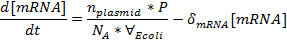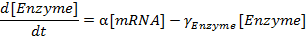Where nplasmid= plasmid copy number in E.coli, P=promoter strength, NA= Avogadaros' Number, VEcoli= cell volume of E.coli, deltamrna=degradation rate of mRNA, alpha= translation rate of mRNA, gammaEnzyme=degradation rate or Enzyme

Combined the two equations, we got the relationship between enzyme concentration and time.Then we combined the equation above with Michaelis-Menten’s equation.